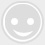# 项链手镯戒指量法(图解)告诉你寸是多长

-回复 -浏览吋即英寸，1英寸=2.54cm
1 尺3 = 16 吋 = 40.64 cm
1 尺5 = 18 吋 = 45.72 cm
1 尺6 = 20 吋 = 50.80 cm
1 尺8 = 22 吋 = 55.88 cm
2 尺0 = 24 吋 = 60.96 cm
2 尺2 = 26 吋 = 66.64 cm